# Machine

The CLS is a 2.9 GeV storage ring with a 12 cell double bend achromat lattice. There are 12 straight sections of equal length. There are two bend magnets per cell and each one is a combined function magnet. One of the main features of a synchrotron storage ring are the number of straight sections, their parameters, and how they are used.

# Storage Ring Parameters

 Lattice Type: Double Bend Achromat Beam Energy: 2.9 GeV Periodicity: 12 cell Circumference: 170.88 m Beam current: 220 mA Momentum Compaction: 0.0038 Straight Length: 5.2 m Straight βx βy, ηx: 8.5m, 4.6m, 0.15m Cavity Frequency: 500 MHz Cavity Voltage: 2.4 MV Harmonic number: 285 Horizontal emittance (εx): 18.1 nm-radian Energy spread (ΔE/E): 0.00111 Bunch Length: 10 mm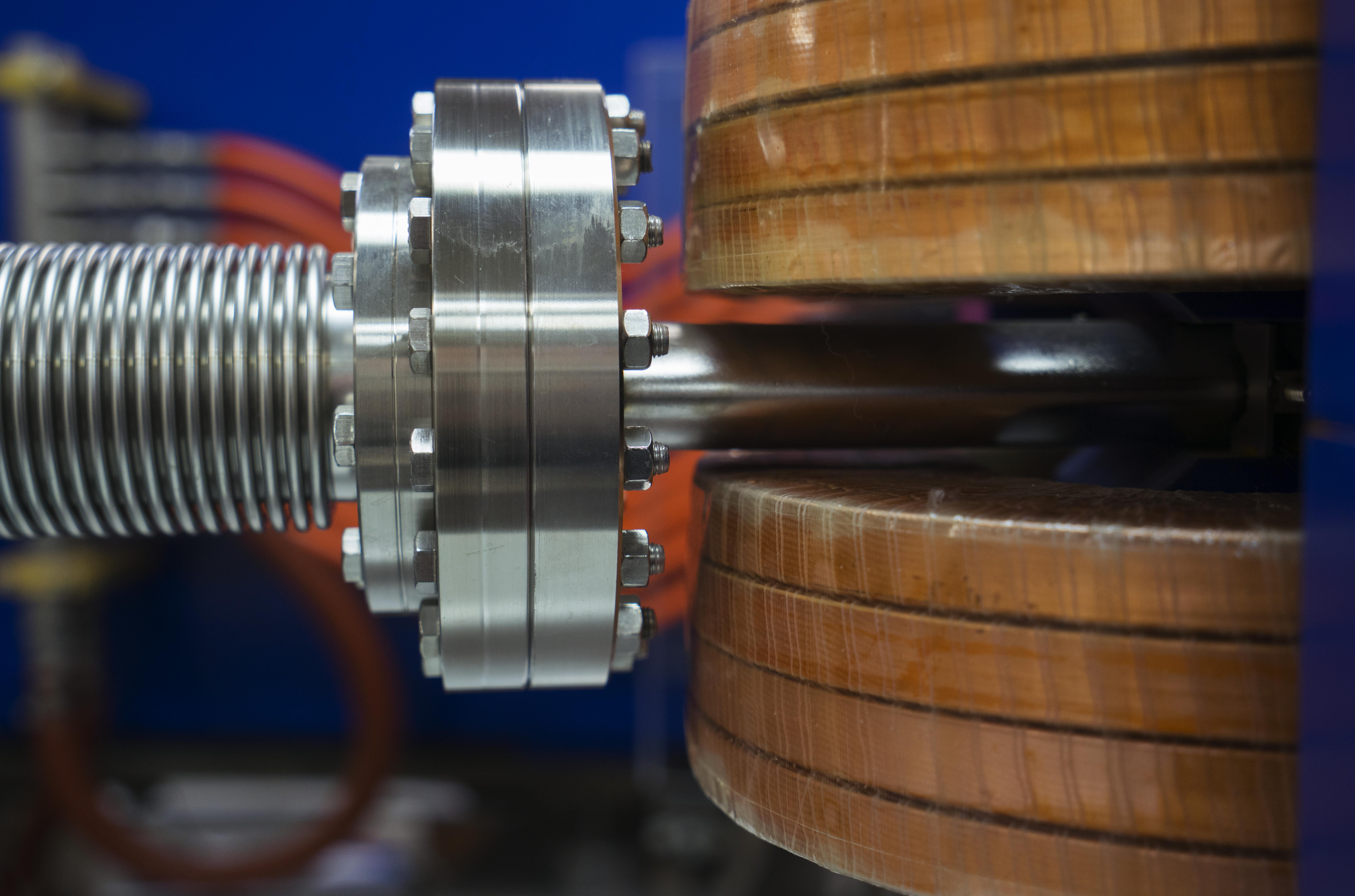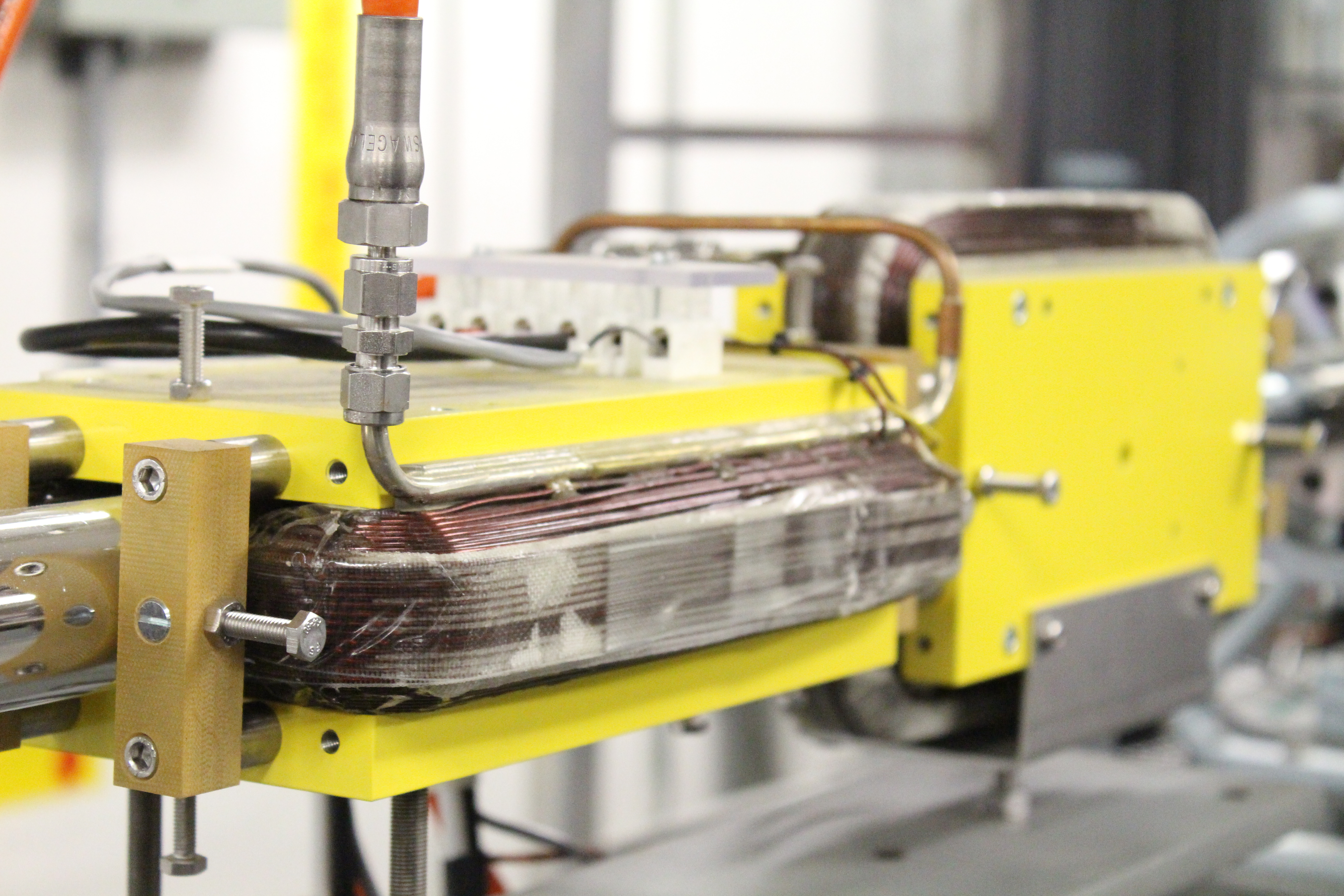The upstream accelerator complex is a 220 keV DC thermionic RF gun. A six section LINAC increases the beam energy from 220 keV to 250 MeV. An energy compression system (3 magnet chicane and RF cavity) compresses the relative beam energy spread by a factor of 10. The beam is then transported into a booster ring and ramped from 250 MeV to 2.9 GeV.

# Straight Section Usage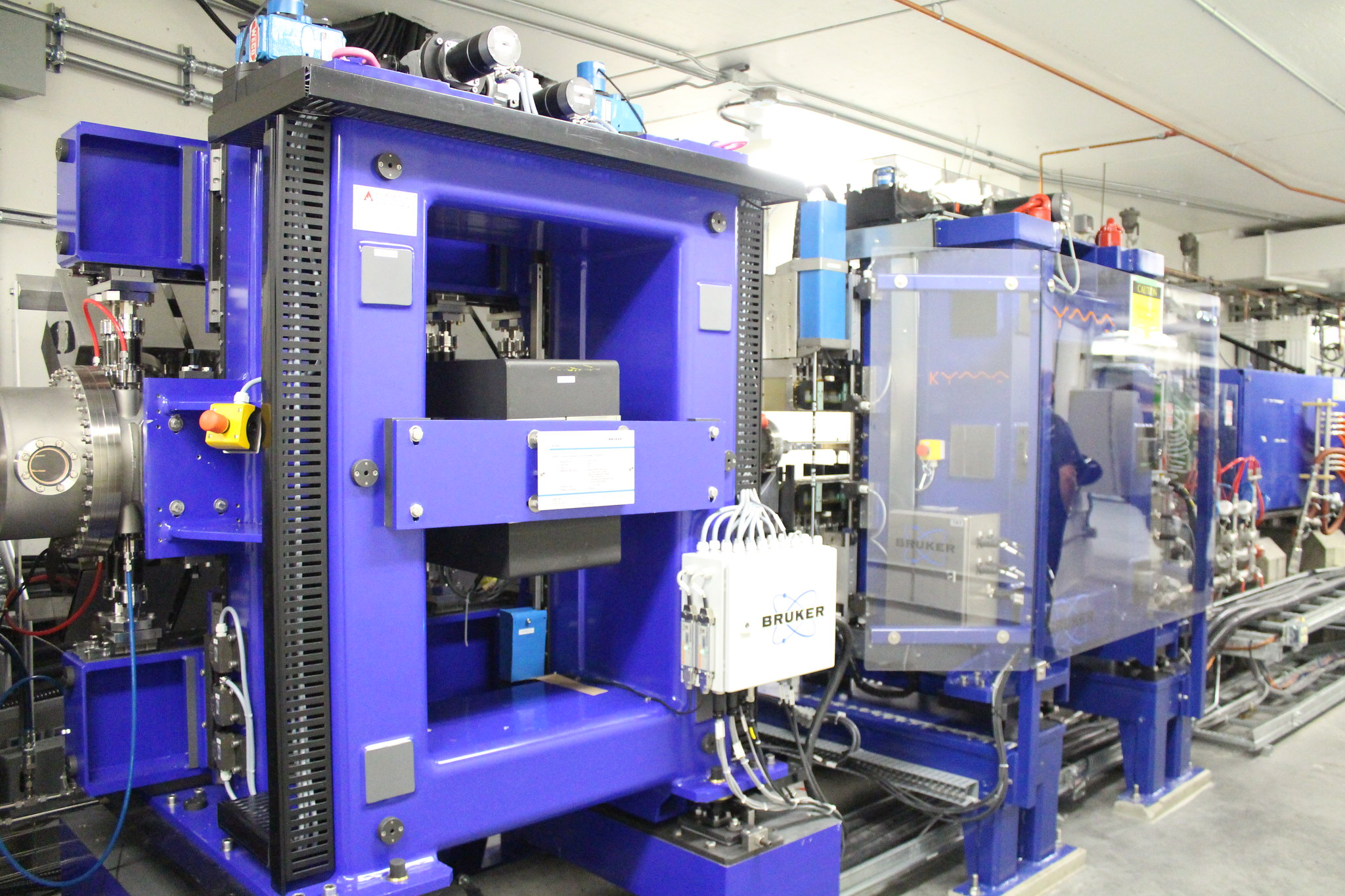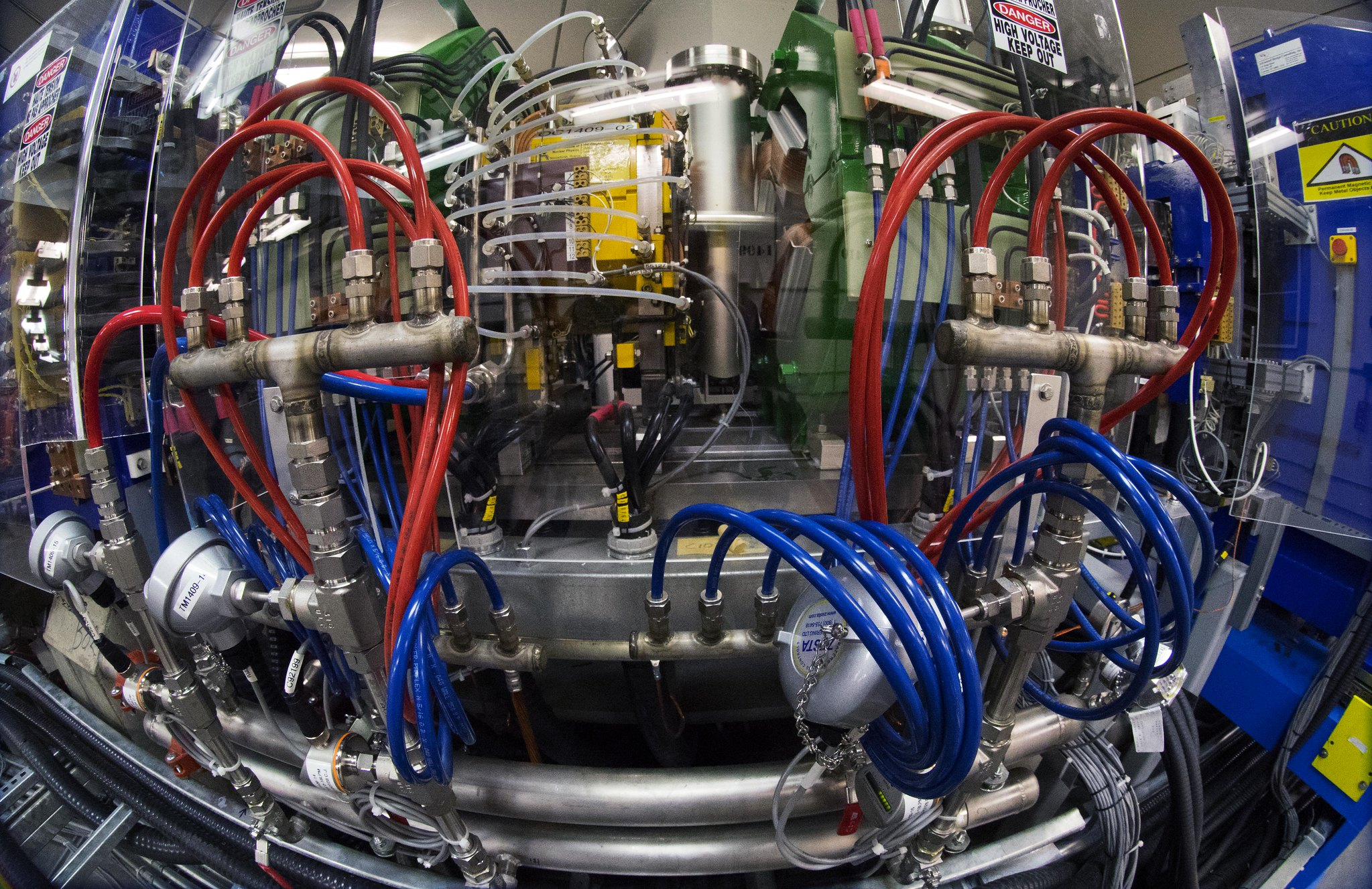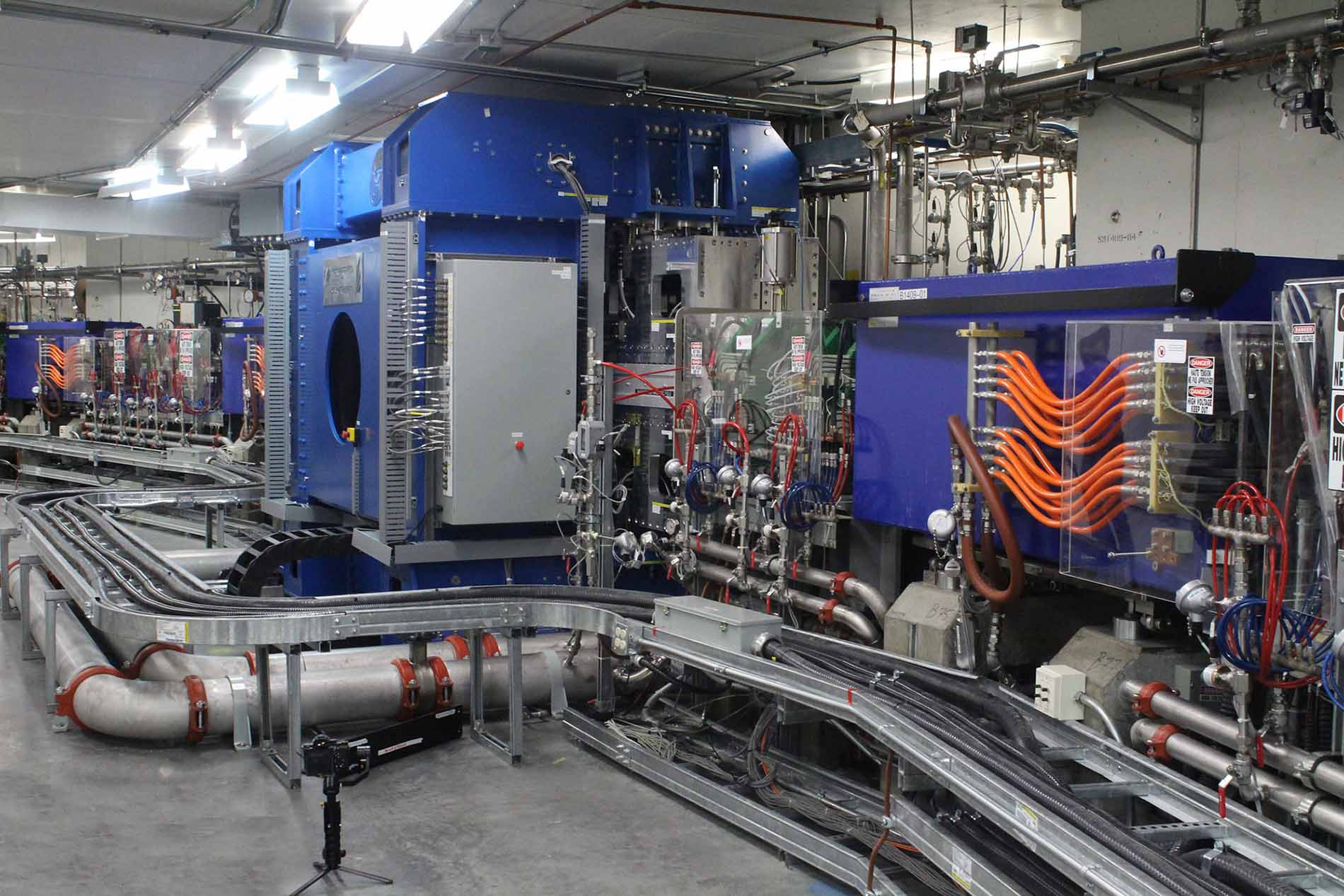Straight Number Device(s) Parameters 1 Injection straight 4 kicker bump 2 Electron beam diagnostic straight - 3 Reserved for future use - 4 Chicaned: In-vacuum undulator In-vacuum wiggler l=20 mm, L=1.582 m, Beff =1.049 T l=80 mm, L=1.28 m, Bpeak =1.948 T 5 Superconducting wiggler l=48 mm, L=0.624 m, Bpeak =4.3 T 6 Superconducting wiggler l=33 mm, L=1.056 m, Bpeak = 2.2 T 7 Chicaned: In-vacuum undulator Out-vacuum wiggler l=18.6 mm, L=1.582 m, Beff =1.016 T l=150 mm, L=1.638 m, Beff =2.103 T 8 In-vacuum undulator l=20 mm, L=1.583 m, Beff =0.959 T 9 Single length dual period APPLE-II undulator l=180 mm, L=3.834 m, Beff =0.697 T l=55 mm, L=3.924 m, Beff =0.877 T 10 Chicaned APPLE-II undulators l=75 mm, L=1.591 m, Beff =0 742 T l=75 mm, L=1.591 m, Beff =0.742 T 11 Chicaned APPLE-II undulators l=45 mm, L=1.189 m, Beff =0.843 T l=185 mm, L=1.635 m, Beff =0.751 T 12 RF Cavity -